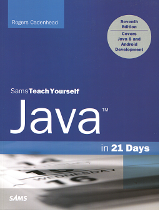# Java in 21 Days (7th Edition) Day 5: Creating Classes and Methods## Notes and Corrections

• View the Certification Practice quiz solution
• Certification answer: Answer (c) is correct. The constructor method of the BiggerValue class calls the method calculateResult(int, int, int). Because this method is not defined in the BigValue class, it must be defined in the BiggerValue class.

Answers (a) is incorrect. It would produce a result equal to 312.0 if the constructor method of the BiggerValue class called calculateResult(int) with the c variable as an argument, but the constructor calls calculateResult(int, int, int).

Answers (b) and (d) are incorrect. Using either of these statements, the example will not compile because the constructor method of the BiggerValue class uses a variable called c which is not defined in the constructor.